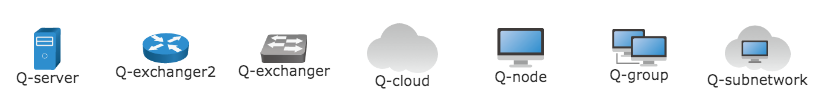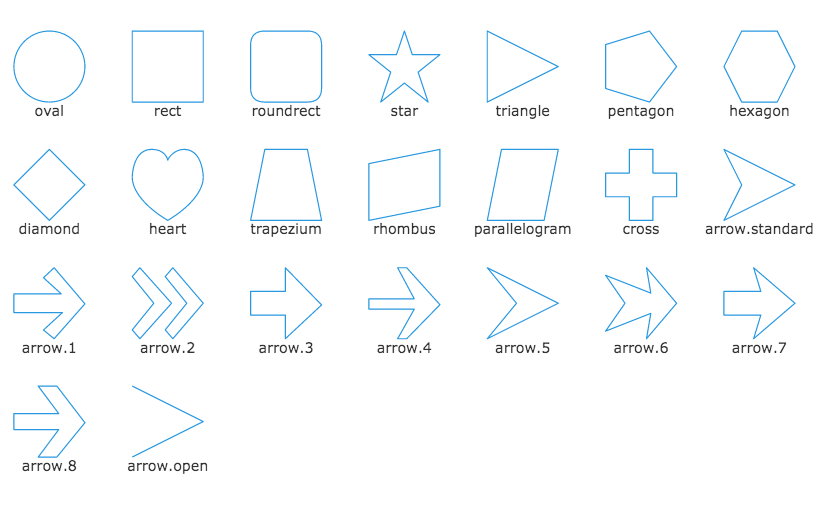Light High Efficiency Graph Component

# Image Registration

Set a name for the resource by registration of images in Qunee. Then set a favourable serialization and source management by this name.

## Register image

Key is the source name. Data is the content of image, which can be image URL, Path object or custom- made draw function, referring to Node Image

``````Q.registerImage = function (key, data){};
``````

### Usage

After image registration, set image property of node by image name

Example

``````var graph = new Q.Graph("canvas");
Q.registerImage('logo', '../demos/images/logo.svg');

var logo = graph.createNode('Logo');
logo.image = 'logo';
``````

Operation effect### Built-in icon

Q.Graphs is configured with some icons, which can be visited via the name of Q-XXX, such as

``````node.image = 'Q-server';
``````

All built-in icons are as follows

``````var x = -300;
for(var n in Q.Graphs){
if(Q.Graphs[n].draw){
var node = graph.createNode('Q-' + n, x, 100);
node.image = 'Q-' + n;
x += 100;
}
}
``````

Operation effect### Built-in images

In Q.Shapes some built-in images are defined and can be used as icons

``````var x = -300;
var y = 200;
var shapes = Q.Shapes.getAllShapes(60, 60);
for(var n in shapes){
var node = graph.createNode(n, x, y);
node.image = shapes[n];
x += 100;
if(x > 300){
x = -300;
y += 100;
}
}
``````

Operation effect